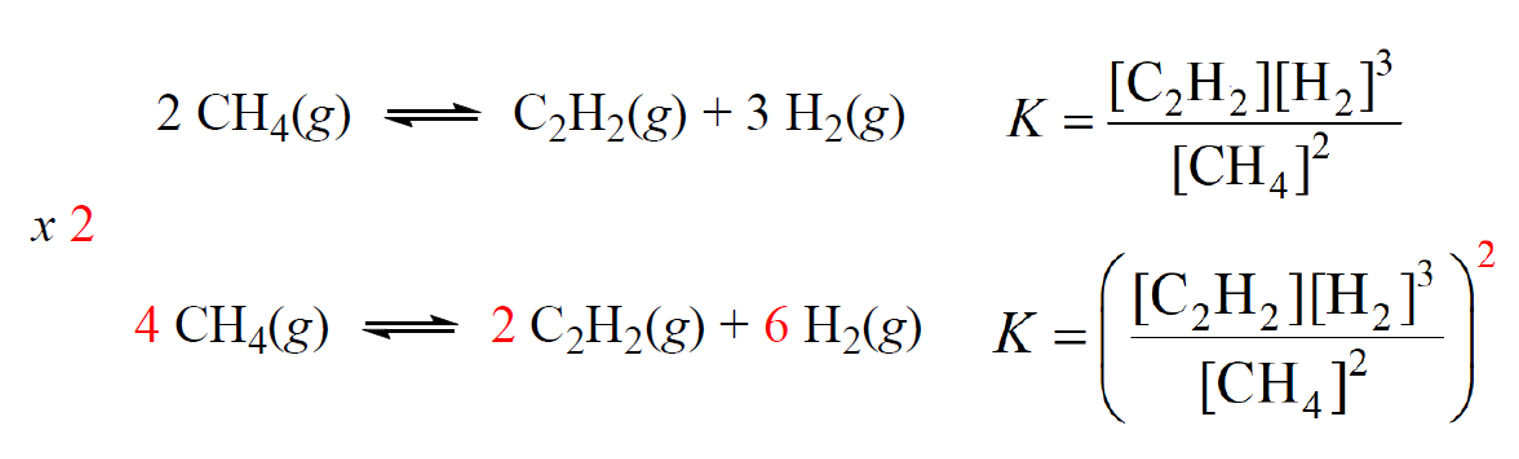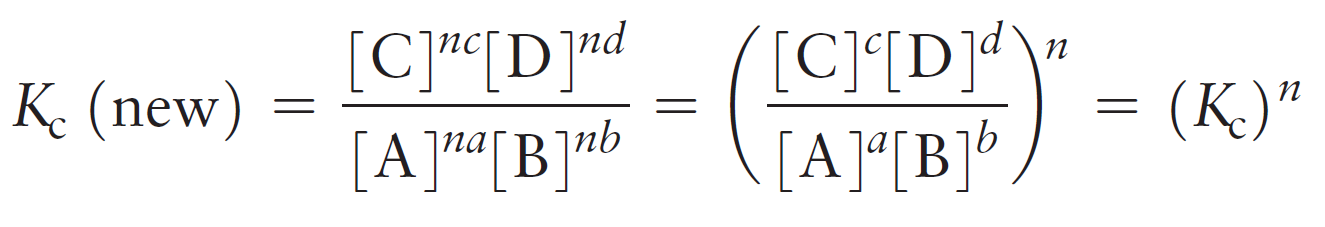## General Chemistry

The value of the equilibrium constant is always given for the specific chemical equation demonstrating the reaction. The direction of the reaction and the coefficients in the balanced chemical equation affect the expression and the value of the equilibrium constant. So, let’s see how the equilibrium constant changes with modifying the chemical equation.

# K for the Reverse Reaction

If the chemical equation is reversed, the equilibrium constant is inverted – it is the reciprocal of the original value of the equilibrium constant.Example:

The equilibrium constant for the decomposition reaction of N2O4 to NO2 is 4.64 x 10-4.

N2O4(g) ⇆ 2NO2(g)

What is the equilibrium constant for the reaction producing N2O4 from NO2?

2NO2(g) ⇆ N2O4(g)

Solution

When a reaction is reversed, then Knew = 1/Koriginal.  Therefore,

Knew = 1/Koriginal= 1/4.64 x 10-4 = 2.16 x 103

# K When the Coefficients are Changed

When the coefficients in the equation are multiplied by any factor, we raise the equilibrium

constant to the same factor.

For example, the second equation for equilibrium between methane and acetylene is multiplied by two, and therefore, the expression for K is raised to the power two:To see how this happens, let’s consider a general chemical equation that is multiplied by a factor of n:

aA + bB ⇆ cC + dD

n (aA + bB) ⇆ n (cC + dD)

The new equilibrium constant is:For example,

The reaction for converting methane to acetylene has an equilibrium constant of K = 0.154 at 2000 K.

2CH4(g) ⇆ C2H2(g) + 3H2(g)

Calculate the equilibrium constant for this process if the reaction is represented as follows:

6CH4(g) ⇆ 3C2H2(g) + 9H2(g)

Solution

Comparing the equation to the original one, we see that it is multiplied by 3. Therefore,

Knew = (Koriginal)= (0.154)3= 0.00365

These principles apply to the equilibrium constant expressed in terms of partial pressure (Kp) and you can find more about it in this article.

Check Also